Math worksheetsCustom math worksheets at your fingertips

# Details for problem "Fraction mult factor has to be filled in"

Quickname: 7056

## Summary

Two fractions are multiplied, one fraction and result given.

## Example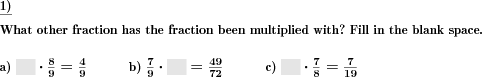## Description

A product term with two fractions is given, as is the result. One of the fractions has been replaced by a blank space. The correct fraction has to be filled in.

The number range for the numerators, denominators can be specified.

Improper fractions can be allowed, disallowed or enforced. In case they may occur, it can be chosen whether they will be output as improper fractions or mixed numbers.

Download free worksheets for this math problem here. There is a second sheet with the solutions. Just click on the respective link.Worksheet 1Solution sheetWorksheet 2Solution sheetWorksheet 3Solution sheet

If you can not see the solution sheets for download, they may be filtered out by an ad blocker that you may have installed. If this is the case, please allow ads for this page and reload the page. The solution sheets will then reappear.

• Do the sample worksheets do not really fit?
• Do you need more math worksheets, with a different level of difficulty?
• Would you like to combine different problems on a worksheet and adjust them to your needs?
• As a teacher, you can put together your own worksheets using the automatically generated math problems provided.
With a free initial credit, you can start creating your own math worksheets in a few minutes.

It does not cost anything to try! Register here, to create custom worksheets now!

## Customization options for this problem

ParameterPossible values
Number of problems1, 2, 3, 4, 5, 6, 7, 8, 9, 10
Number range10, 20, 30, 40, 50, 100, 200, 500, 1000, 10000, 100000
Improper fractionsNo, Yes, as a fraction, Yes, as a mixed number

## Similar problems

without blanks, a plain multiplication of two fractions problemMultiply two fractions.Multiply fractions

## Other types of problems that appear on worksheets with this problem:

RelevanceNameDescriptionQuicknameExample
****Fractions add subAdding and subtracting fractions6408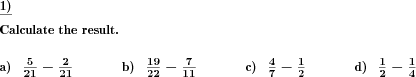****Dividing fractions by whole numbersA fraction has to be divided by a whole number.5220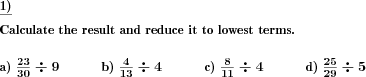****Missing elements in unlike fractions In unlike fractions of the same value, numerators or denominators have to be filled in.6652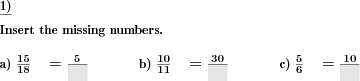****Fractions order relationsInsert the correct relational symbol between two fractions1433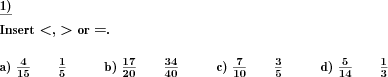Deutsche Version dieser Aufgabe
These informational pages with samples describe math problems that can be combined on custom math worksheets with solutions for home and school use.